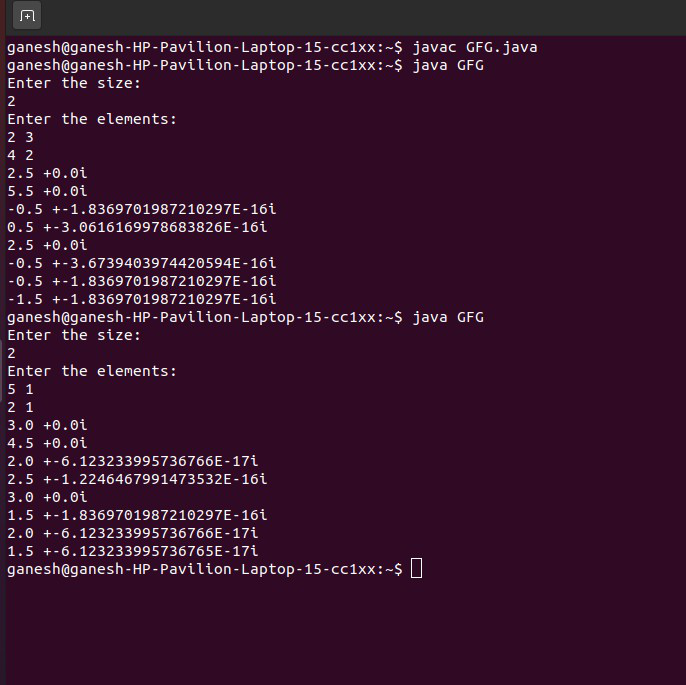Open in App
Not now

# How to Perform a 2D FFT Inplace Given a Complex 2D Array in Java?

• Difficulty Level : Hard
• Last Updated : 28 Jul, 2022

A fast Fourier transform (FFT) is an algorithm to compute the discrete Fourier transform (DFT) and its inverse. Fourier analysis converts time (or space) to the frequency and vice versa. FFT reduces the computation time required to compute a discrete Fourier Transform and improves the performance by a factor 100 or more over direct evaluation of DFT.  FFT computes such transformations by factorizing the DFT Matrix into a product of sparse.

Here we use a 2D array which will help us find the Fast Fourier Transform.  This Algorithm is useful in pattern recognition.

Examples

Input:

Enter the size:

2

Enter the  elements:

2  3

4  2

Output:

2.5 + 0.0 i

5.5 + 0.0 i

-0.5 + -1.8369701987210297E-16 i

0.5 + -3.0616169978683826E-16 i

2.5 + 0.0 i

-0.5 + -3.6739403974420594E-16 i

-0.5 + -1.8369701987210297E-16 i

-1.5 + -1.8369701987210297E-16 i

Input:

Enter the size:

2

Enter the elements :

5 1

2 1

Output:

3.0 + 0.0 i

4.5 + 0.0 i

2.0 + -6.123233995736766E-17 i

2.5 + -1.2246467991473532E-16 i

3.0 + 0.0 i

1.5 + -1.8369701987210297E-16 i

2.0 + -6.123233995736766E-17 i

1.5 + -6.123233995736765E-17 i

Approach:

• Enter the size of the array.
• We will take 4 arrays of data type double named input, realOut, imaginary.
• Give the input of the 2D array.
• Now the let us call a function dft, which will help us to calculate
• Now, we will calculate the height and width of the input data.
• Now, let us iterate the loop height and width,
• Now to calculate the DFT we would get it in terms of exponential, which can be converted into cosine and sine terms, which are labeled as real and imaginary parts. These can be calculated by using these formulas.
• Iterating it with height and width we calculate realOut, using the formula:

realOut[y][x]+=(input[y1][x1]*Math.cos(2*Math.PI*((1.0*x*x1/width)+(1.0*y*y1/height))))/Math.sqrt(width*height);

• Similarly, we will get the imaginary output using this formula:

imagOut[y][x]-=(input[y1][x1]*Math.sin(2*Math.PI*((1.0*x*x1/width)+(1.0*y*y1/height))))/Math.sqrt(width*height);

• Now, we would print these values in the form of  a+ib.

Example:

## Java

 `// Java program to perform  a 2D FFT Inplace Given a Complex``// 2D Array` `// Declare the needed libraries``import` `java.io.*;``import` `java.util.Scanner;` `public` `class` `GFG {``    ``public` `static` `void` `main(String[] args)``    ``{``        ``// enter the size of the matrix``        ``System.out.println(``"Enter the size:"``);` `        ``// declaring the scan element``        ``Scanner sc = ``new` `Scanner(System.in);``        ``// scan the size of the matrix``        ``int` `n = sc.nextInt();` `        ``// Declaring the matrices in double datatype``        ``// Declaring the input variable where we take in the``        ``// input``        ``double``[][] input = ``new` `double``[n][n];` `        ``// Taking the matrices for real value``        ``double``[][] realOut = ``new` `double``[n][n];` `        ``// Taking the matrices for imaginary output``        ``double``[][] imagOut = ``new` `double``[n][n];` `        ``// Enter the values of elements of the DFT Matrix``        ``System.out.println(``"Enter the elements:"``);` `        ``// Taking the input of the array``        ``// By iterating the two loops``        ``for` `(``int` `i = ``0``; i < n; i++) {``            ``for` `(``int` `j = ``0``; j < n; j++) {``                ``input[i][j] = sc.nextDouble();``            ``}``        ``}` `        ``// Calling the function discrete``        ``discrete(input, realOut, imagOut);` `        ``// Closing  the function scanner``        ``sc.close();``    ``}` `    ``// Now by taking the discrete function``    ``// This is the declaration of the function``    ``// This function includes 4 parameters``    ``// The parameters are the 4 matrices.``    ``static` `void` `discrete(``double``[][] input,``                         ``double``[][] realOut,``                         ``double``[][] imagOut)``    ``{` `        ``// Height is the variable of data type int``        ``// the length of the input variable is stored in``        ``// variable height``        ``int` `height = input.length;` `        ``// The input of the first index length is stored in``        ``// variable width``        ``int` `width = input[``0``].length;` `        ``// Iterating the input till height stored in``        ``// variable y``        ``for` `(``int` `y = ``0``; y < height; y++) {` `            ``// Taking the input iterating till width in``            ``// variable x``            ``for` `(``int` `x = ``0``; x < width; x++) {` `                ``// Taking another variable y1 which will be``                ``// the continuation of``                ``// the variable y``                ``// This y1 will be iterating till height``                ``// This index of the variable starts at 0``                ``for` `(``int` `y1 = ``0``; y1 < height; y1++) {` `                    ``// This index x1 iterates till width``                    ``// This x1 is continuation of x``                    ``// The variables y1 and x1 are the``                    ``// continuation of summable of x and y``                    ``for` `(``int` `x1 = ``0``; x1 < width; x1++) {` `                        ``// realOut is the variable which``                        ``// lets us know the real output as``                        ``// we do the summation of exponential``                        ``// signal``                        ``// we get cos as real term and sin``                        ``// as imaginary term``                        ``// so taking the consideration of``                        ``// above properties we write the``                        ``// formula of real as``                        ``// summing till x and y and``                        ``// multiplying it with cos2pie``                        ``// and then dividing it with width``                        ``// *height gives us the real term``                        ``realOut[y][x]``                            ``+= (input[y1][x1]``                                ``* Math.cos(``                                    ``2` `* Math.PI``                                    ``* ((``1.0` `* x * x1``                                        ``/ width)``                                       ``+ (``1.0` `* y * y1``                                          ``/ height))))``                               ``/ Math.sqrt(width * height);` `                        ``// Now imagOut is the imaginary term``                        ``// That is the sine term``                        ``// This sine term can be obtained``                        ``// using sin2pie and then we divide``                        ``// it using width*height The``                        ``// formulae is same as real` `                        ``imagOut[y][x]``                            ``-= (input[y1][x1]``                                ``* Math.sin(``                                    ``2` `* Math.PI``                                    ``* ((``1.0` `* x * x1``                                        ``/ width)``                                       ``+ (``1.0` `* y * y1``                                          ``/ height))))``                               ``/ Math.sqrt(width * height);``                    ``}` `                    ``// Now we will print the value of``                    ``// realOut and imaginary outputn The``                    ``// ppoutput of imaginary output will end``                    ``// with value 'i'.``                    ``System.out.println(realOut[y][x] + ``" +"``                                       ``+ imagOut[y][x]``                                       ``+ ``"i"``);``                ``}``            ``}``        ``}``    ``}``}`

Output:My Personal Notes arrow_drop_up